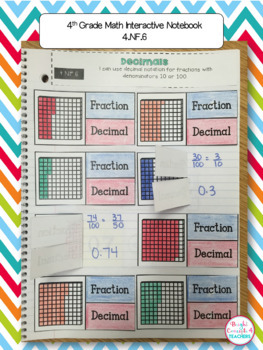# 4th Grade Math Interactive Notebook {Common Core Aligned}Subject
Resource Type
File Type

PDF

(29 MB|172 pages)
Standards
• Product Description
• Standards

Interactive notebooks are a great learning tool in the classroom. They serve as a resource for reviewing standards, a reference tool, or even as an assessment.

This notebook covers all the 4th Grade Common Core MATH standards (including Operations & Algebraic Thinking, Numbers and Operations in Base 10, Geometry and Measurement and Data, Number and Operations-Fractions)

This product includes:

-Notebook cover

-Divider tabs

-Strand Dividers

-Pictures of EVERY page so you can have an example of how each activity can be used

-Student friendly "I can" statements at the top of each page.

I know your students will love making these notebooks as a method to reinforce all the CCSS math standards they have been taught.

Skills covered:

Operations and Algebraic Thinking:

-Interpreting multiplication

-Meanings for multiplication

-Problem solving using multiplication

-Problem solving using missing factors

-Problem solving using division

-Problem solving with 3-digit numbers

-Multi-step word problems

-Using variable equations to solve word problems

-Prime v. composite numbers

-Factors

-Problem solving with factors

-Problem solving with prime v. composite numbers

-Number patterns

-Shape patterns

Numbers and Operations in Base 10:

-Place Value

-Base 10, Number Names & Expanded Form

-Greater than, less than, equal to

-Rounding

-Adding and Subtracting multi-digit whole numbers

-Multiplication up to 4-digits

-Multiplication Problem Solving

-Division with and without remainders

-Division Problem Solving

Geometry:

-Identify and draw points, lines, line segments, rays, angles, perpendicular and parallel lines

-Triangles: right, acute and obtuse

-Triangles: equilateral, isosceles, scalene

-Quadrilaterals: identify parallel and perpendicular lines

-Lines of symmetry

Measurement and Data:

-Measurement Units

-Measurement Conversion Tables

-Distance Word Problems

-Time Word Problems

-Volume Word Problems

-Money Word Problems

-Perimeter of rectangles

-Area of squares and rectangles

-Perimeter of rectangles

-Area Word Problems

-Area v. Perimeter Word Problems

-Line Plots

-Angles and Circles

-Measuring Angles with a Circle

-Measuring Angles with a Protractor

-Solving for Unknown Angles

Number and Operations-Fractions

-Equivalent Fractions

-Comparing Fractions

-Common Denominator

-Decomposing Fractions

-Fraction Word Problems (Adding & Subtracting)

-Multiply Fractions & Whole Numbers

-Fraction Word Problems (Multiplication)

-Equivalent Fractions with Denominator as 10 and 100

-Decimals

-Comparing Decimals

More Math Interactive Notebooks:

KINDERGARTEN Math Interactive Notebook

***************************************************

If you would like to get updates on NEW & CURRENT resources...

**************************************************

Email me at brightconcepts4teachers@gmail.com if you have any questions!

Compare two decimals to hundredths by reasoning about their size. Recognize that comparisons are valid only when the two decimals refer to the same whole. Record the results of comparisons with the symbols >, =, or <, and justify the conclusions, e.g., by using a visual model.
Use decimal notation for fractions with denominators 10 or 100. For example, rewrite 0.62 as 62/100; describe a length as 0.62 meters; locate 0.62 on a number line diagram.
Express a fraction with denominator 10 as an equivalent fraction with denominator 100, and use this technique to add two fractions with respective denominators 10 and 100. For example, express 3/10 as 30/100, and add 3/10 + 4/100 = 34/100.
Recognize a line of symmetry for a two-dimensional figure as a line across the figure such that the figure can be folded along the line into matching parts. Identify line-symmetric figures and draw lines of symmetry.
Classify two-dimensional figures based on the presence or absence of parallel or perpendicular lines, or the presence or absence of angles of a specified size. Recognize right triangles as a category, and identify right triangles.
Total Pages
172 pages
N/A
Teaching Duration
1 Year
Report this Resource to TpT
Reported resources will be reviewed by our team. Report this resource to let us know if this resource violates TpT’s content guidelines.